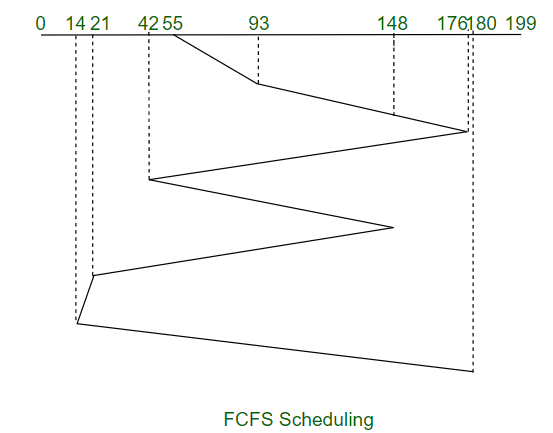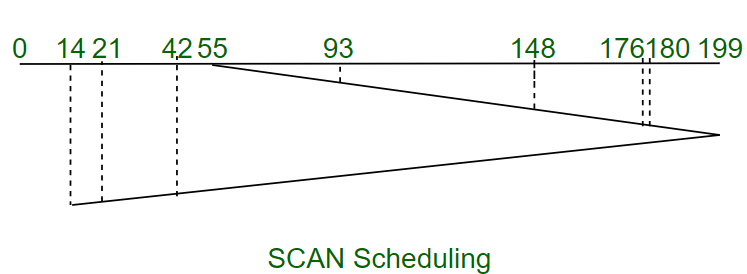Open In App

# Difference between FCFS and SCAN disk scheduling algorithms

FCFS disk scheduling algorithm:
As the name suggests, the FCFS Scheduling Algorithm processes requests in the sequential order in which they arrive in the disk queue. Even if a higher priority request arrives in the schedule later, FCFS will process the request in the order that they have arrived and hence we can say that FCFS has a fair policy.

Example:
Consider a disk with 200 tracks (0-199) and the disk queue having I/O requests in the following order as follows: 93, 176, 42, 148, 21, 14, 180. The current head position of the Read\Write head is 55. Calculate the total number of track movements of Read/Write head using FCFS.Total Seek Time,

```= (93-55) + (176-93) + (176-42)
+ (148-42) + (148-21)
+ (21-14) + (180-14)
= 661  ```

SCAN disk scheduling algorithm:
As the name suggests, the SCAN Algorithm scans all the tracks of the disk in a back and forth manner such that it will first process all the requests in one direction till it reaches the last track in that direction then it reverses the direction and starts servicing the requests in it comes across. This algorithm is also known as Elevator Algorithm since it works like an elevator by continuously moving in one direction servicing requests and then reversing direction.

Example:
Consider a disk with 200 tracks (0-199) and the disk queue having I/O requests in the following order as follows: 93, 176, 42, 148, 21, 14, 180. The current head position of the Read\Write head is 55. Calculate the total number of track movements of Read/Write head using SCAN.Total Seek Time,

```= (199-55) + (199-14)
= 329  ```

Now, let’s see the difference between FCFS and SCAN disk scheduling algorithms:

Sr.No.FCFS Disk Scheduling AlgorithmSCAN Disk Scheduling Algorithm
1.The FCFS Scheduling Algorithm will processes requests in the sequential order in which they arrive in the disk queue.The SCAN Scheduling Algorithm will first process the requests in one direction till it reaches the end of the disk then the disk arm reverses the direction and starts servicing the requests in it opposite order.
2.FCFS algorithm has a fair policy as requests processed as per schedule so there are less chances of indefinite postponement.It may happen that the location of the request was just visited by the disk arm so the request will have a long waiting time.
3.FCFS algorithm gives the lowest throughput among all the disk scheduling algorithms.SCAN algorithm has a better throughput than FCFS scheduling algorithm.
4.The average seek time of FCFS algorithm is the highest among all the disk scheduling algorithms as it does not try to optimize the seek time.The average seek time of SCAN algorithm is much lower as compared to FCFS scheduling algorithm.